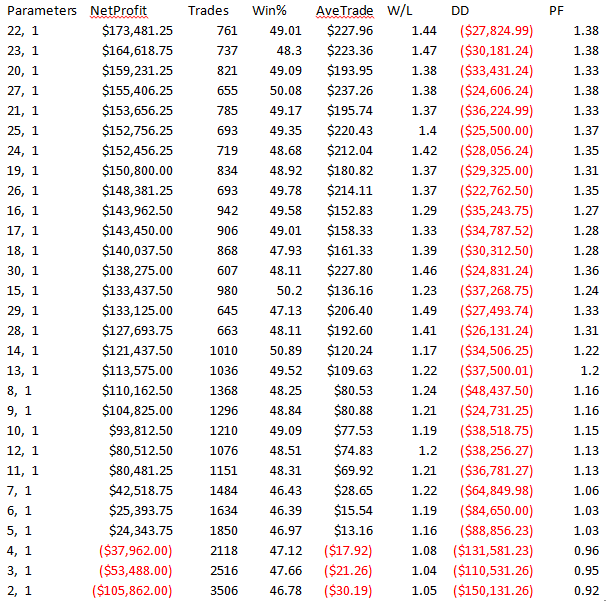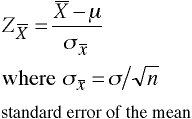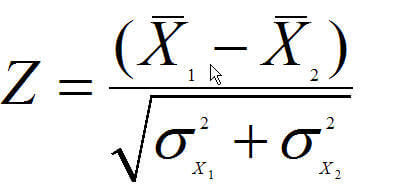# Development of Intermarket Trading SystemsIn my past article, Intermarket Is Fundamentally Sound,  I covered some of the basic premises and history of intermarket trading systems. While the previous entry was more theoretical, this article is more practical. Indeed, I will be discussing how intermarket analysis can be used to generate mechanical signals. I will also walk you through the process I followed in developing and improving my own intermarket analysis mechanical trading methods.

### First Generation Intermarket Trading Systems

Let’s begin our study by taking a look at what I call the “first generation” intermarket systems. These basically used intermarket forces as a filter. Our analysis will be done as follows: futures will use one lot and be compared with some market. For example, we will use Treasury bonds and compare utility stocks to the DJ Bond index. Measures like CARG are controlled by account size and sizing. If we are not trading a portfolio then sizing to normalize risk volatility is not needed and it simplifies our results. If we do this analysis on stocks or ETFs as the markets we are trading, then we need to look at these calculations because dollar analysis is meaningless as it depends when gains occur. In futures, it’s always the same dollar value per point, except for major contract changes like 1997 in the S&P500.

Our first generation system is very simple and is as follows:

Sub IntermarketDummy(SLen,Relate)
Dim IntOsc As BarArray
IntOsc=Close Of independent1-Average(Close Of independent1,SLen,0)
If Relate=1 Then
If IntOsc<0 Then Sell("",1,0,Day,Market)
End If
If Relate<>1 Then
If IntOsc>0 Then Sell("",1,0,Day,Market)
End If
End Sub

If you’re wondering how this trading model code was generated, you’ll be interested to know it’s based upon the free Intermarket Divergence TradeStation Tool. You can download a free copy at the conclusion of this article

Let’s now look at our first example. We will use the UTY (Philadelphia electrical utility average) and trade U.S. thirty year treasury bonds (24 hour session, continuous contract). Utility stocks are positively correlated so relate = 1. We will use \$50 for slippage and commission, and optimize the moving average lookback value from 2-30 in steps of 1, using the date range 09/22/1987 to 04/10/2015.In our first simple model, we can see that the results are not very good. Only one set of parameters (the top set) made any money on the short side (how do you know this?). In the bond market, due to the strong upward bias, most systems do not make any money on the short side. Does the use of UTY in this way predict bonds? In order to figure out this question, we would need to compare to a standard sample. This will be a simple price crossover system which we will then do a Z-test to see if UTY is predictive. Our standard system which is a bogey test is as follows:

Sub InterBogeyDummy(SLen)
Dim IntOsc As BarArray
IntOsc=Close-Average(Close,SLen,0)
If IntOsc<0 Then Sell("",1,0,Day,Market)
End Sub

We will use the same date range. We will also optimize over the same date range and use the same values for the moving average 2-30. Finally, we are also using \$50.00 slippage and commission, as we did before.We can obviously see that using UTY was a big improvement to our results even though the results of the basic intermarket system are not that good. Albeit it’s extremely obvious just by glancing at the two tables that using UTY did certainly help, I will walk through the Z-test to show this fact. Indeed, it is always important to run statistical analyses on your systems to confirm that they are really performing better than we could expect just by chance. We did not run in sample and out of sample tests because our only goal here was to show that UTY was predictive of Treasury bonds.

### The Z-Test

The goal of constructing a statistical distribution is to compare some observed phenomena with another observed phenomena, and see if they are the same or different. The Z-test is an easy way to compare two types of distributions and determine, with some quantifiable degree of certainty, that they are different.

The Z-test makes use of one of the fundamental tenants of statistical theory:

The error in the mean is calculated by dividing the dispersion by the square root of the number of data points.

The error in the mean can be thought of as a measure of how reliable a mean value is. The more samples you have the more reliable the mean is. However, this reliability is directly related to the square root of the number of samples that you have. If you wanted to improve the reliability by a factor of 10, for example, you would have to get 100 times the number of samples. This can be difficult to do sometimes!

Comparing two sample means is easy. To find the difference of the two sample means in units of sample mean error, use the following formula:But for comparing two samples directly, one needs to compute the Z statistic in the following manner:Where

• Xis the mean value of sample one
• X2 is the mean value of sample two
• σx1 is the standard deviation of sample one divided by the square root of the number of data points
• σx2 is the standard deviation of sample two divided by the square root of the number of data points

Here is a specific example of the Z-test application (in very simple, non-trading terms):

Eugene vs. Seattle rainfall comparison over 25 years (so N = number of samples = 25):

Eugene

Seattle

mean=51.5 inches

mean=39.5 inches

dispersion=8.0

dispersion=7.0

N=25

N=25

error in mean = 8.0/5

error in mean = 7.0/5

error in mean = 1.6

error in mean 1.4

So far this example:

• X1= 51.5
• X2= 39.5
• X1– X= 12
• σx1= 1.6
• σx2= 1.4
• sqrt of σx12+ σx22 =sqrt(1.62 + 1.42) = sqrt(2.56 +1.96) = 2.1

Therefore, the Z statistic is 12/2.1 = 6 which means there is a highly significant difference between these two distributions. This means it really does rain significantly more in Eugene than in Seattle.

You can verify these numbers by using the Z-test tool (comparing two means) accessible from the statistical tools area.

Probability

z value

0.25

0.7

* this is often used in in power calculations power=0.75, β=0.25

0.2

0.85

* this is often used in in power calculations power=0.8, β=0.2

0.1

1.29

Cell

0.05

1.65

* this is often used for testing statistical significant p<0.05

0.025

1.96

* this is often used to define the 95% confidence interval (2.5% each side)

0.01

2.33

Cell

0.005

2.58

CellNow that we’ve taken a look at the non-trading example, let’s walk through our trading example that we had earlier. We will look at the net profit column and see if they come from a different distribution.

In a perfect world, we would like to see Z of at least 1.65 but in the trading world with all the noise and small samples, Z values are often not this high. The worst thing we can do is make a type II error.

For reference, a type II error occurs when you accept the null hypothesis when it is false. In this case, it would be concluding that there are no significant differences between the two samples when they actually are different. In trading, this is tantamount to having a statistically good system and throwing it away because you don’t see that due to an error in the calculations!

We will do our calculations as follows. We will optimize across a range of parameters and will drop the lowest 10% and the top 10% rounded up. Let’s consider these to be potential outliers. We will then use the results from above, with 30 combinations for both. We will drop the best two and the worst two for both sets of data.

Our results are as follows:

Average Bogey

-\$60,671.8

Std deviation Bogey

69072.74

Intermarket Average

\$111,670.8

Intermarket Std deviation

50652.47

Ave Diff

\$172,342.5

sqr of std deviation

85654.63

Z-test

2.012063

This is significant at the 97.8% level. Put another way, we can say with 97.8% certainty that using UTY does help us predict and trade the 30-year bonds.

We also want to see if we have a probability that the system is going to be profitable. The way to do that is to compare the average net profit over the optimization space to the standard deviation of that space. We want a ratio of at least 1.0 for the system to be considered stable with positive expectations over the space. In our case, the ratio is 2.20 which is a good sign!

-- Murray Ruggiero of Using EasyLanguage

Also see...

PART 1: The Road to Finding Intermarket-Based Turning Points
PART 2: Intermarket Analysis is Fundamentally Sound

Quickly Build Intermarket Trading Systems in EasyLanguage

### About the Author Murray Ruggiero

•Alonso says:

“This is significant at the 97.8% level. Put another way, we can say with 97.8% certainty that using UTY does help us predict and trade the 30-year bonds.”

But you are comparing a negative mean to a positive mean. What do you expect? If you select a bad bogey the result will always be good. In reality you have only shown that using UTY is better than some random crossover and nothing else.

“You can verify these numbers by using the Z-test tool (comparing two means) accessible from the statistical tools area.”

Copy and paste? This is not funny.